FormalPara Key Points for Decision Makers
 The minimum sample size needed for a discrete-choice experiment (DCE) depends on the specific hypotheses to be tested. DCE practitioners should realize that a small size effect may still be meaningful, but that a limited sample size prevents detection of such small effects. Policy makers should not make a decision on non-significant outcomes without considering whether the study had a reasonable power to detect the anticipated outcome.

## 1 Introduction

Discrete-choice experiments (DCEs) have become a commonly used instrument in health economics and patient-preference analysis, addressing a wide range of policy questions [1, 2]. DCEs allow for a quantitative elicitation of individuals’ preferences for health care interventions, services, or policies. The DCE approach combines consumer theory , random utility theory , experimental design theory , and econometric analysis . See Louviere et al. , Hensher et al. , Rose and Bliemer , Lancsar and Louviere , and Ryan et al.  for further details on conducting a DCE.

DCE-based research in health care is often concerned about establishing the impact of certain healthcare interventions and aspects (i.e., attributes) thereof on patients’ decisions . Consequently, a typical research question is to establish whether or not individuals are indifferent between two attribute levels. For instance: Do patients prefer delivery at home more than in a hospital?; Do patients prefer a medical specialist over an nurse practitioner?; Do patients prefer every 5 year screening over every 10 year screening?; Do patients prefer a weekly oral medication over a monthly injection?; Do patients prefer the explanation of their medical results through a face-to-face contact more than through a letter? As a result, an important design question is the size of the sample needed to answer such a research question. When considering the required sample size, DCE practitioners need to be confident that they have sufficient statistical power to detect a difference in preferences when this difference is sufficiently large. A practical solution (that does not require any sample size calculations) is to simply maximize the sample size given the research budget at hand, i.e., trying to overpower the study as much as possible. This is beneficial for reasons other than statistical precision (e.g. to facilitate in-depth analysis). However, particularly in the health care area, the number of eligible patients and healthcare professionals is generally limited. Although theory exists as to the calculation of sample size requirements for stated choice data, it does not address the issue of minimum sample size requirements in terms of testing for specific hypotheses based on the parameter estimates produced .

The purpose of this paper is threefold. The first objective is to provide insight into whether and how researchers have dealt with sample size calculations for health care-related DCE studies. The second objective is to introduce and explain the required sample size for parameter estimates in DCEs. The final objective of this manuscript is to provide a step-by-step guide for the calculation of the minimum sample size requirements for DCEs in healthcare.

## 2 Literature Review

### 2.1 Methods

To gain insight into the current approaches to sample size determination, we reviewed health care-related DCE studies published in 2012. Older literature was ignored, as the research frontier for methodological issues has shifted a lot over the past years [1, 22]. MEDLINE was used to identify healthcare-related DCE studies, replicating the methodology of two comprehensive reviews of the healthcare DCE literature [1, 2]. The following search terms were used: conjoint, conjoint analysis, conjoint measurement, conjoint studies, conjoint choice experiment, part-worth utilities, functional measurement, paired comparisons, pairwise choices, discrete choice experiment, dce, discrete choice mode(l)ling, discrete choice conjoint experiment, and stated preference. Studies were included if they were choice-based, published as a full-text English language article, and applied to healthcare. Consideration was given to background information of the studies, and detailed consideration was given to whether and how sample size calculations were conducted. We also briefly describe the methods that have been used to obtain sample size estimates so far.

### 2.2 Literature Review Results

The search generated 505 possible references. After reading abstracts or full articles, 69 references met the inclusion criteria. The appendix shows the full list of references [Electronic Supplementary Material (ESM) 1]. Table 1 summarizes the review data. Most DCE studies were from the UK, with the USA, Canada, and Australia also major contributors. Studies having 4–6 attributes and 9–16 choice sets per respondent were commonly used in the published healthcare-related DCE studies in 2012. The sample sizes differed substantially between the DCE studies.

Of 69 DCEs, 22 (32 %) had sample sizes smaller than 100 respondents, whereas 16 (23 %) of the 69 DCEs had sample sizes larger than 600 respondents; six (9 %) DCEs even had sample sizes larger than 1000 respondents. More than 70 % of the DCE studies (49 of 69) did not (clearly) report whether and what kind of sample size method was used; 12 % of the studies (8 of 69) just referred to other DCE studies to explain the sample size used. For example, Huicho et al.  mentioned that “Based on the experience of previous studies [24, 25], we aimed for a sample size of 80 nurses and midwives”, and Bridges et al.  mentioned “In a previously published pilot study, the conjoint analysis approach was shown to be both feasible and functional in a very low sample size (n = 20) ”. In 13 % of the DCE studies (9 of 69 ), one or more of the following rules of thumb were used to estimate the minimum sample size required: that proposed by (1) Johnson and Orme [37, 38]; (2) Pearmain et al. ; and/or (3) Lancsar and Louviere .

In short, the rule of thumb as proposed by Johnson and Orme [37, 38] suggests that the sample size required for the main effects depends on the number of choice tasks (t), the number of alternatives (a), and the number of analysis cells (c) according to the following equation:

$$N > 500c/(t \times a)$$
(1)

When considering main effects, ‘c’ is equal to the largest number of levels for any of the attributes. When considering all two-way interactions, ‘c’ is equal to the largest product of levels of any two attributes .

The rule of thumb proposed by Pearmain et al.  suggests that, for DCE designs, sample sizes over 100 are able to provide a basis for modeling preference data, whereas Lancsar and Louviere  mentioned “our empirical experience is that one rarely requires more than 20 respondents per questionnaire version to estimate reliable models, but undertaking significant post hoc analysis to identify and estimate co-variate effects invariably requires larger sample size”.

Four of 69 (6 %) reviewed DCE studies used a parametric approach to estimate the minimum sample size required (a parametric approach can be used if one assumes, for example based on the law of large numbers, that the focal quantity—an estimated probability or coefficient—is Normally distributed. This assumption facilitates the derivation of the minimum sample sizes required). That is, three studies used the parametric approach as proposed by Louviere et al.  and one study  reported the parametric approach as proposed by Rose and Bliemer . Louviere et al.  assume the study is being conducted to measure a choice probability with some desired level of accuracy. The asymptotic sampling distribution (i.e., the distribution as sample size N → ∞) of a proportion p N, obtained by a random sample of size N, is Normal with mean p (the true population proportion) and variance pq/N, where q = 1−p. The minimum sample size to estimate the true proportion within α 1 % of the true value p with a probability α 2 or greater has to satisfy the requirement that Prob(|p Np| ≤ α1 p) ≥ α 2, which can be calculated using the following equation:

$$N > (q/(rpa_{1}^{2} )) \cdot \left( {\varPhi^{ - 1} (1 - \frac{{\alpha_{2} }}{2})} \right)^{2}$$
(2)

where Φ −1 is the inverse cumulative Normal distribution function, and r is the number of choice sets per respondent. Hence, the parametric approach as proposed by Louviere et al.  suggests that the sample size required for the main effects depends on the number of choice sets per respondent (r), the true population proportion (p), the one minus true population proportion (q), the inverse cumulative Normal distribution function (Φ −1), the allowed deviation from the true population proportion (α 1), and the significance level (α 2).

The parametric approach that has been recently introduced by Rose and Bliemer  focuses on the minimum sample size required based on the most critical parameter (i.e., to be able to determine whether each parameter value is statistically significant from zero). This parametric approach can only be used if prior parameter estimates are available and not equal to zero. The minimum required sample size to state with 95 % certainty that a parameter estimate is different from zero can be determined according to the following equation:

$$N > \max_{k} (1.96\sqrt {\sum\nolimits_{\gamma k} / } \gamma k)^{2}$$
(3)

where γ k is the parameter estimate of attribute k, and Σ γk is the corresponding variance of the parameter estimate of attribute k.

### 2.3 Comment on the State of Play

The disadvantage of using one of the rules of thumb mentioned in paragraph 2.2 is that such rules are not intended to be strictly accurate or reliable. The parametric approach as proposed by Louviere et al.  is not suitable for determining the minimum required sample size for coefficients in DCEs, as this approach focuses on choice probabilities and does not address the issue of minimum sample size requirements in terms of testing for specific hypotheses based on the parameter estimates produced. The parametric approach for minimum sample size calculation proposed by Rose and Bliemer  is solely based on the most critical parameter, so it is not specific to a certain hypothesis. It also does not depend on a desired power level for the hypothesis tests of interest.

## 3 Determining Required Sample Sizes for Discrete-Choice Experiments (DCEs): Theory

In this section we explain the analysis needed to determine the minimum sample size requirements in terms of testing for specific hypotheses for coefficients in DCEs. Our proposed approach is more general than the parametric approaches mentioned in Sect. 2, as it can be used for any particular hypothesis that is relevant to the researcher. We outline which elements are required before such a minimum sample size can be determined, why these elements are needed, and how to calculate the required sample size. To provide a step-by-step guide that is useful for researchers from all different kinds of backgrounds, we strive to keep the number of formulas in this section as low as possible. Nevertheless, a comprehensive explanation of the minimum sample size calculation for coefficients in DCEs can be found in the appendix (ESM 2).

### 3.1 Required Elements for Estimating Minimum Sample Size

Before the minimum sample size for coefficients in a DCE can be calculated, the following five elements are needed:

• Significance level (α)

• Statistical power level (1−β)

• Statistical model used in the DCE analysis [e.g., multinomial logit (MNL) model, mixed logit (MIXL) model, generalized multinomial logit (G-MNL) model]

• Initial belief about the parameter values

• The DCE design.

#### 3.1.1 Significance Level (α)

The significance level α sets the probability for an incorrect rejection of a true null hypothesis. For example, if one wants to be 95 % confident that the null hypothesis will not be rejected when it is true, α needs to be set at 1−0.95 = 0.05 (i.e. 5 %). Conversely, if one decides to perform a hypothesis test at a 1−α confidence level, there is by definition an α probability of finding a significant deviation when there is in fact no true effect. Perhaps unsurprisingly, the smaller the imposed value of α (i.e., the more certainty one requires), the larger the minimum required sample size will be.

#### 3.1.2 Statistical Power Level (1−β)

β indicates the probability of failing to reject a null hypothesis when the null hypothesis is actually false. The chosen value of beta is related to the statistical power of a test (which is defined as 1−β). As we want to assess whether a parameter value (coefficient) is significantly different from zero, we can define the sample size that enables us to find a significant deviation from zero in at least (1−β) × 100 % of the cases. For example, a statistical power of 0.8 (or 80 %) means that a study (when conducted repeatedly over time) is likely to produce a statistically significant result eight times out of ten. A larger statistical power level will increase the minimum sample size needed.

#### 3.1.3 Statistical Model Used in the DCE Analysis

The calculation of the minimum required sample size also depends on the type of statistical model that will be used to analyze the DCE data (e.g., MNL, MIXL, G-MNL). The type of statistical model affects the number of parameters that needs to be estimated, the corresponding parameter values, and the parameter interpretation. As a consequence, the estimation precision of the parameters, which we will characterize through the variance covariance matrix of the estimated parameters, also depends on the statistical model that is used. In order to properly determine the estimation precision of each of the parameters, the statistical model needs to be specified.

#### 3.1.4 Initial Belief About the Parameter Values

Of course, if the true values of the parameters (coefficients) were known, one would not need to execute the DCE. Nevertheless, before a minimum sample size can be determined, an initial estimate of the parameter values is required for two reasons. First, in models that are nonlinear in the parameters, such as choice models, the asymptotic variance–covariance matrix (AVC) depends on the values of the parameters themselves. This AVC is an intermediate stage in the sample size calculation (see Sect. 3.2 for more details), and reflects the expected accuracy of the statistical estimates obtained using the statistical model as identified under Sect. 3.1.3. Second, before a power calculation can be done, one has to describe a specific hypothesis and the power one wants to achieve given a certain degree of misspecification (i.e., the degree to which the true coefficient value deviates from its hypothesized value). As null hypothesis, we will use the hypothesis that there is no influence so the coefficient equals zero. The initial estimate of the parameter value can then be used as value for the effect size. The closer to zero the effect size is, the more difficult it will be to find a significant effect and hence the larger the minimum sample size will be. To obtain some insight into these parameter values, a small pilot DCE study—for example with 20–40 respondents—may be helpful.

#### 3.1.5 DCE Design

The large literature on efficient design generation indicates the importance of the design in getting accurate estimates and powerful tests. The DCE design is described by the number of choice sets, the number of alternatives per choice set, the number of attributes, and the combination of the attribute levels in each choice set. The DCE design has a direct influence on the AVC, which affects the estimation precision of the parameters, and hence will have a direct influence on the minimum sample size required.Footnote 1

### 3.2 Sample Size Calculation for DCEs

Once all five required elements mentioned in Sect. 3.1 have been determined, the minimum required sample size for the estimated coefficients in a DCE can be calculated. First, as an intermediate part of the sample size calculation, the AVC has to be established. That is, the statistical model (Sect. 3.1.3), the initial belief on the parameter values, denoted with γ (Sect. 3.1.4), and the DCE design (Sect. 3.1.5), are all needed to infer the AVC matrix, $$\sum_{\gamma }$$, of the estimated parameters. Details on how to construct the variance–covariance matrix from this information can be found, for example, in McFadden  for MNL and in Bliemer and Rose  for panel MIXL. A variance–covariance matrix is a square matrix that contains the variances and covariances associated with all the estimated coefficients. The diagonal elements of this matrix contain the variances of the estimated coefficients, and the off-diagonal elements capture the covariances between all possible pairs of coefficients. For hypothesis tests on individual coefficients, we only need the diagonal elements of $$\sum_{\gamma }$$, which we denote by Σγk for the kth diagonal element.

Once the AVC, $$\sum_{\gamma }$$, of the estimated parameters has been established and the confidence level (α), the power level (1−β), and the effect sizes (δ) are set, the minimum required sample size (N) for the estimated coefficients in a DCE can be calculated (see Eq. 4).

$$N > ((z_{1 - \beta } + z_{1 - \alpha } )\sqrt {\sum_{\gamma k} } /\delta )^{2}$$
(4)

Each of the elements in this sample size calculation intuitively makes sense. In particular, with a larger effect size δ, a smaller sample size (N) will suffice to have enough power to find a significant deviation. Testing at a higher confidence level α increases z 1−α ,Footnote 2 and thus increases the minimum required sample size (N). The same holds when more statistical power is desired, as this increases z 1−β .Footnote 3 When the variance-covariance matrix contains smaller variance ($$\sum_{{\gamma {\text{k}}}}$$) the minimum sample size (N) required decreases, as the estimates will be more precise. Smaller values for $$\sum_{{\gamma {\text{k}}}}$$ can be obtained from using more choice sets, more alternatives per choice set or a more efficient design.

## 4 Determining Required Sample Sizes for DCEs: A Practical Example

In this section, a practical example is provided to explain, step-by-step, how the minimum sample size requirement for a DCE study can be calculated. This is illustrated using R-code, which can also be found at http://www.erim.eur.nl/ecmc.

The DCE study used for this illustration concerns a DCE about patients’ preferences for preventive osteoporosis drug treatment . In this DCE study, patients had to choose between drug treatment alternatives that differed in five treatment attributes: route of drug administration, effectiveness, side effects (nausea), treatment duration, and out-of-pocket costs. The DCE design was orthogonal and contained 16 choice sets. Each choice set consisted of two unlabeled drug treatment alternatives and an opt-out option.

In what follows, we show in seven steps how the minimum sample size for coefficients can be calculated for the DCE on patients’ preferences for preventive osteoporosis drug treatment.

1. Step 1

Significance Level (α)

We first have to set the confidence through α. In the illustration, we choose α = 0.05. The resulting confidence level is 95 %, assuming a one-tailed testFootnote 4 (Box 1)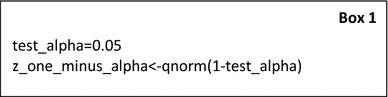2. Step 2

Statistical Power Level (1−β)

The second step is to choose the statistical power level. For our illustration, we opt for a standard statistical power level of 80 % (i.e., β = 0.20, hence 1−β = 0.80) (Box 2).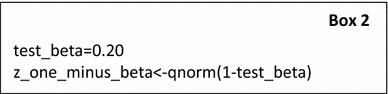3. Step 3

Statistical Model Used in the DCE Analysis

The third step is to choose the statistical model to analyze the DCE data. For our illustration, we opt for an MNL model. In the R code, this affects the way the AVC needs to be calculated, which is outlined in step 6

4. Step 4

Initial Belief About the Parameter Values

The fourth step concerns the initial beliefs about the parameter values. The DCE illustration regarding patients’ preferences for preventive osteoporosis drug treatment contains five attributes (two categorical attributes and three linear attributes) , resulting in eight parameters to be estimated (see Table 2 column ‘parameter label’). We use the point estimates of the parameters as our guess of the coefficients and the effect sizes δ (see Table 2 column ‘initial belief parameter value’) (Box 3)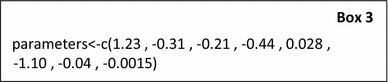5. Step 5

The DCE design

The fifth step focuses on the DCE design. The DCE design requires eight parameters to be estimated (ncoefficients = 8). Each choice set contains three alternatives (nalts = 3); that is, two drug treatment alternatives, and one opt-out alternative. The DCE design contains 16 choice sets (nchoices = 16) (Box 4)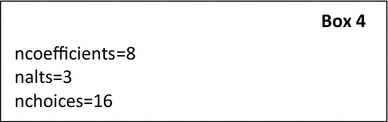• The DCE design should be coded in a text-file in such a way that it can be read correctly into R. That is, the DCE design should contain one row for each alternative. So, there should be nalts × nchoices rows (see Table 3 as an example for our illustration, which contains 48 rows (i.e., 3 alternatives × 16 choice sets); rows 1–3 correspond to choice set 1, rows 4–6 correspond to choice set 2, etc.)

Each row should contain the coded attribute levels for that alternative. See Table 3 for how the DCE design for our illustration was coded (columns A–F). For example, row 1 corresponds to the first preventive drug treatment alternative in choice set 1: a drug treatment alternative (value 1, column A) that should be taken as a tablet every week (value 1, column B1), which will result in a 5 % reduction of a hip fracture (value 5, column C) without side effects (value 0, column D), for which the drug treatment duration will be 10 years (value 10, column E) and out-of-pocket costs of €120 are required (value 120, column F). Be aware that only the DCE design (i.e., the ‘white part’ of Table 3) should be in a text file, so that it can be read correctly in R (Box 5)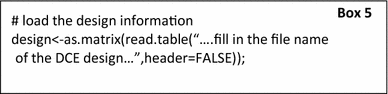6. Step 6

Estimation Accuracy

Having our statistical model, our initial beliefs about the parameter values (i.e., our guess of the effect sizes) and our DCE design matrix, we are able to compute the AVC matrix ($$\sum_{\gamma }$$) (Box 6)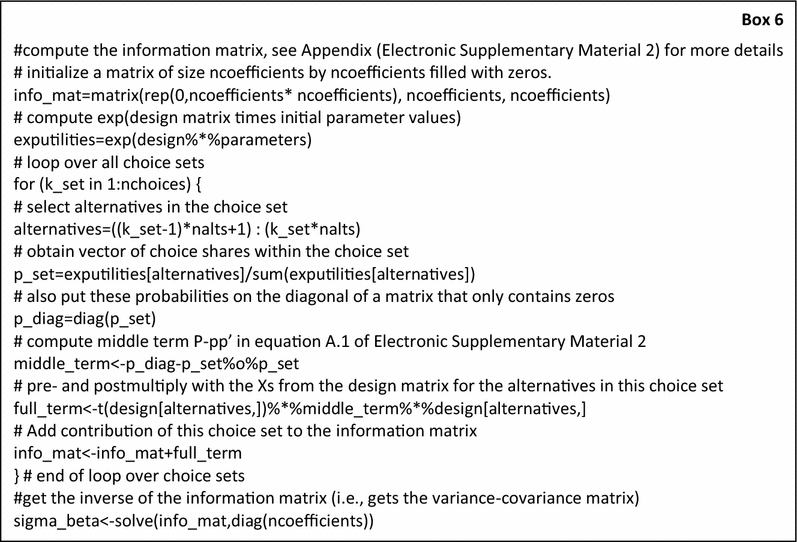7. Step 7

Sample Size Calculation

The final step is to calculate the required sample size for the MNL coefficients in our DCE. Hereto we use Eq. 4 (Box 7)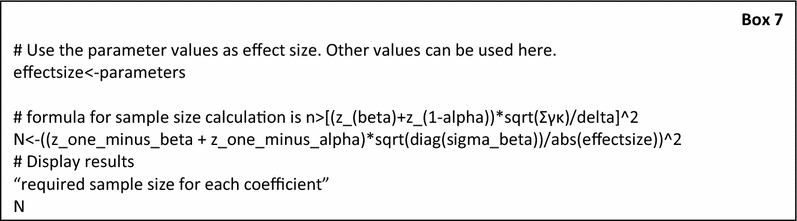• The results of the minimum sample size required to obtain the desired power level for finding an effect when testing at a specific confidence level for each parameter are shown in Table 4. To illustrate the impact of the probability that we will find a significant effect given a specific effect size, we also computed the required sample size for the statistical power level 1−β equal to 0.6, 0.7, and 0.9. Additionally, we also computed the required sample sizes assuming a significance level α of 0.1, 0.025, and 0.01

As can be seen from Table 4, one needs a minimum sample size of 190 respondents with a statistical power of 0.8 and assuming an α = 0.05, whether ‘injection every 4 months’ is significantly different from ‘tablet once a month (reference attribute level)’ (Table 4, column B2). If a smaller sample size of, for example, 111 respondents were to be used and no significant result to be found for this parameter, one has a statistical power of 0.6, assuming an α = 0.05, to conclude that respondents do not prefer ‘tablet every month’ over ‘injection every 4 months’. As a proof of principle, we compared the standard errors and confidence intervals from the actual study  against the predicted standard errors and confidence intervals. The results showed that they were quite similar (Table 5), which gives further evidence that our sample size calculation makes sense.

## 5 Discussion

In this paper, we have summarized how researchers have dealt with sample size calculations for health care-related DCE studies. We found that more than 70 % of the health care-related DCE studies published in 2012 did not (clearly) report whether and what kind of sample size method was used. Just 6 % of the health care-related DCE studies published in 2012 used a parametric approach for sample size estimation. Nevertheless, the parametric approaches used were not suitable as a power calculation for determining the minimum required sample size for hypothesis testing for coefficients based on DCEs. To fill in this gap, we explained the analysis needed to determine the required sample size in DCEs from a hypothesis testing perspective. That is, we clarified that the following five elements are needed before such a minimum sample size can be determined: significance level (α), statistical power level (1−β), statistical model used in the DCE analysis, initial belief about the parameter values, and the DCE design. An important feature of the resulting sample size formula is that the required sample size tends to grow exponentially. For example, when one wants a certain power level to detect an effect that is 50 % smaller, the required sample will be four times larger.

To build a bridge between theory and practice, we created a generic R-code as a practical tool for researchers to be able to determine the minimum required sample size for coefficients in DCEs. We then illustrate step-by-step how the sample size requirement can be obtained using our R-code. Although the R-code presented in this paper is for MNL only, the theory is also suitable for other choice models, such as the nested logit, mixed logit, scaled-MNL, or generalized-MNL.

Our approach for determining the minimum required sample size for coefficients in DCEs can also be extended to functions of parameters. For example, one might want to know whether patients are willing to pay a specific amount to increase effectiveness by 10 %. In order to test such a hypothesis, confidence intervals for a willingness-to-pay measure are needed. Once how these will be inferred from the limiting distribution of the parameters  is determined, ΣWTP (instead of Σγ) is known and the required sample size can be computed.

From a practical point of view, in health care-related DCEs, the number of patients and physicians that can be approached is often given, and sometimes rather small. Especially in these cases, our tool could indicate that power will be low. Using efficient designs (striving for small values for $$\sum_{{\gamma {\text{k}}}}$$), more alternatives per choice set, or clear wording and layout are ways to increase the power that is achieved.

The approach presented in this paper can also be used to reverse engineer the power that a specific design has for a given sample size. This can help researchers who find an insignificant result to ensure that they had sufficient power to detect a reasonably sized effect.

## 6 Conclusion

The use of sample size calculations for healthcare-related DCE studies is largely lacking. We have shown how sample size calculations can be conducted for DCEs when researchers are interested in testing whether a particular attribute (level) affects the choices that patients or physicians make. Such sample size calculations should be executed far more often than is currently the case in healthcare, as under-powered studies may lead to false insights and incorrect decisions for policy makers.